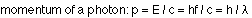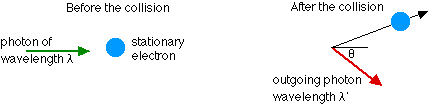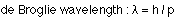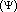## Wave-particle duality

8-4-00

Sections 27.4 - 27.6

### The Compton effect

Although photons have no mass, they do have momentum, given by:Convincing evidence for the fact that photons have momentum can be seen when a photon collides with a stationary electron. Some of the energy and momentum is transferred to the electron (this is known as the Compton effect), but both energy and momentum are conserved in such a collision. Applying the principles of conservation of energy and momentum to this collision, one can show that the wavelength of the outgoing photon is related to the wavelength of the incident photon by the equation:### Wave-particle duality

To explain some aspects of light behavior, such as interference and diffraction, you treat it as a wave, and to explain other aspects you treat light as being made up of particles. Light exhibits wave-particle duality, because it exhibits properties of both waves and particles.

Wave-particle duality is not confined to light, however. Everything exhibits wave-particle duality, everything from electrons to baseballs. The behavior of relatively large objects, like baseballs, is dominated by their particle nature; to explain the behavior of very small things like electrons, both the wave properties and particle properties have to be considered. Electrons, for example, exhibit the same kind of interference pattern as light does when they're incident on a double slit.

### The de Broglie wavelength

In 1923, Louis de Broglie predicted that since light exhibited both wave and particle behavior, particles should also. He proposed that all particles have a wavelength given by:Note that this is the same equation that applies to photons.

de Broglie's prediction was shown to be true when beams of electrons and neutrons were directed at crystals and diffraction patterns were seen. This is evidence of the wave properties of these particles.

Everything has a wavelength, but the wave properties of matter are only observable for very small objects. If you work out the wavelength of a moving baseball, for instance, you will find that the wavelength is far too small to be observable.

### What is a particle wave?

The probability of finding a particle at a particular location, then, is related to the wave associated with the particle. The larger the amplitude of the wave at a particular point, the larger the probability that the electron will be found there. Similarly, the smaller the amplitude the smaller the probability. In fact, the probability is proportional to the square of the amplitude of the wave.

### Quantum mechanics

All these ideas, that for very small particles both particle and wave properties are important, and that particle energies are quantized, only taking on discrete values, are the cornerstones of quantum mechanics. In quantum mechanics we often talk about the wave functionof a particle; the wave function is the wave discussed above, with the probability of finding the particle in a particular location being proportional to the square of the amplitude of the wave function.